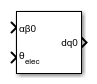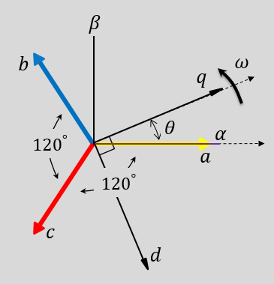# Clarke to Park Angle Transform

Implement αβ0 to dq0 transform

• Library:
• Simscape / Electrical / Control / Mathematical Transforms

•## Description

The Clarke to Park Angle Transform block converts the alpha, beta, and zero components in a stationary reference frame to direct, quadrature, and zero components in a rotating reference frame. For balanced three-phase systems, the zero components are equal to zero.

You can configure the block to align the phase a-axis of the three-phase system to either the q- or d-axis of the rotating reference frame at time, t = 0. The figures show the direction of the magnetic axes of the stator windings in the three-phase system, a stationary αβ0 reference frame, and a rotating dq0 reference frame where:

• The a-axis and the q-axis are initially aligned.• The a-axis and the d-axis are initially aligned.In both cases, the angle θ = ωt, where

• θ is the angle between the a and q axes for the q-axis alignment or the angle between the a and d axes for the d-axis alignment.

• ω is the rotational speed of the d-q reference frame.

• t is the time, in s, from the initial alignment.

The figures show the time-response of the individual components of equivalent balanced αβ0 and dq0 for an:

• Alignment of the a-phase vector to the q-axis• Alignment of the a-phase vector to the d-axis### Equations

The Clarke to Park Angle Transform block implements the transform for an a-phase to q-axis alignment as

`$\left[\begin{array}{c}d\\ q\\ 0\end{array}\right]=\left[\begin{array}{ccc}\text{sin}\left(\theta \right)& -\text{cos}\left(\theta \right)& 0\\ \text{cos}\left(\theta \right)& \text{sin}\left(\theta \right)& 0\\ 0& 0& 1\end{array}\right]\left[\begin{array}{c}\alpha \\ \beta \\ 0\end{array}\right]$`

where:

• α and β are the alpha-axis and beta-axis components of the two-phase system in the stationary reference frame.

• 0 is the zero component.

• d and q are the direct-axis and quadrature-axis components of the two-axis system in the rotating reference frame.

For an a-phase to d-axis alignment, the block implements the transform using this equation:

`$\left[\begin{array}{c}d\\ q\\ 0\end{array}\right]=\left[\begin{array}{ccc}\text{cos}\left(\theta \right)& \text{sin}\left(\theta \right)& 0\\ -\text{sin}\left(\theta \right)& \text{cos}\left(\theta \right)& 0\\ 0& 0& 1\end{array}\right]\left[\begin{array}{c}\alpha \\ \beta \\ 0\end{array}\right]$`

## Ports

### Input

expand all

Alpha-axis, α, beta-axis, β, and zero components of the two-phase system in the stationary reference frame.

Data Types: `single` | `double`

Angular position of the rotating reference frame. The value of this parameter is equal to the polar distance from the vector of the a-phase in the abc reference frame to the initially aligned axis of the dq0 reference frame.

Data Types: `single` | `double`

### Output

expand all

Direct-axis and quadrature-axis components and the zero component of the system in the rotating reference frame.

Data Types: `single` | `double`

## Parameters

expand all

Align the a-phase vector of the abc reference frame to the d- or q-axis of the rotating reference frame.

 Krause, P., O. Wasynczuk, S. D. Sudhoff, and S. Pekarek. Analysis of Electric Machinery and Drive Systems. Piscatawy, NJ: Wiley-IEEE Press, 2013.